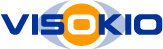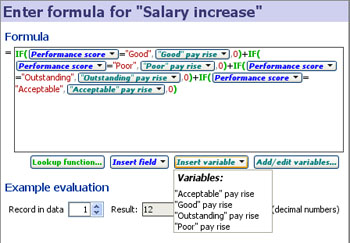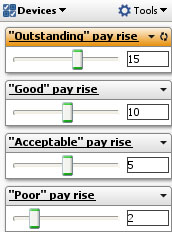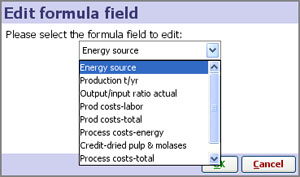# Using Formula Fields and Variables

### Creating new columns calculated by formulae and user-managed variable assumptions

Note: See also examples of commonly-used formulae, or consult the Functions Guide for documentation of all functions available for use in formulae.

Omniscope allows you to add formula fields, which are special fields (columns) whose values are calculated and re-calculated on opening/refresh according to the defined formula using inbuilt functions. Formulae combine references to other fields, mathematical expressions, a standard set of functions similar to spreadsheets such as Excel, and additional Omniscope-specific Subset functions which operate on evaluated subsets of your overall data set. Formulae in Omniscope are written in a similar sytax to spreadsheet formulae, using an interactive, visual editor and some easy-to-use variables for defining assumptions and sensitivity ranges. Subset functions do not exist in spreadsheets and therefore have their own syntax unique to Omniscope.

Formulas are composed and edited using Omniscope's powerful Omniscope Formula editor which provides error feedback, preview of partial results and function assistance. Omniscope also allows you to define additional values called Variables, which are input assumptions, rather than values found within the fields (columns) of the data set. Variables have a single value, but are usually presented in a device allowing the user to change the value across a configurable range (see Add/Edit variables below). Variables are used for enabling multi-variate, dynamic sensitivity analyses and real-time modelling options that can be controlled by any user of the file.

### Formulae Editor Example

This example below shows a formula for calculating staff salary increases based on performance scores using a conditional IF statement to test a field text value and apply the corresponding Variables:This example is taken from the embedded demo file File > Demos > Human resources, tab: Salary cost analysis. The data includes a column called 'Performance score' with 4 values; '"Acceptable", "Good", "Outstanding" and "Poor". The formula above uses nested IF functions (in green) and compares the value in the field'Performance score' (in blue), with typed in test values (enclosed in double quotes) reflecting the names of the Variables already defined, such as "Good" (in red). If the values match, i.e. an employee's value in the 'Performance score' column is "Good", then they will receive the pay rise defined by the Variable 'Good Pay rise'(since all the other IF comparisons will fail and the other parts of the expression will be zero). The Variable 'Good pay rise' has a default value (10) and a range. Each Variable defined also has an associated Side Bar device. If you reveal the Variables on the Side Bar, users of the file can perform real-time modelling and sensitivity analysis just by changing the assumed values using the sliders (see above right).

Unlike formulae in spreadsheets based on individual cell references, Omniscope formulae only contain field references, which are equivalent to saying "the cell in column X in the same row". You cannot define an Omniscope formula that depends on the value in a cell belonging to another record (row).

## Managing formulae

Formula fields can be added and defined using the Formula Editor accessible from the Main Toolbar using Data > Formulas and also from within the Table View using the Formulas button.

Use Data > Formulas > Add formula field  to create a new field whose values calculated using a formula.  You will be prompted to enter the name of the new field, before seeing the Formula editor which you use to edit your formula.### Editing an existing formula

To edit an existing formula, choose Data > Formulas > Edit formula.  You will first be prompted to choose the formula field.Alternatively, use the field menu to edit the formula for a given field.  Field menus can be found by right-clicking the Table View column header, right-clicking the Sidebar filter device title, or through the Data> Manage Fields window. The Formula Editor will open showing your existing formula which can be edited as text.

### Converting a field to a formula field

If you already have a blank field with the correct name, right-click the column header in the Table View, or use the Edit option in Data > Manage fields.  From the menu, choose Change to formula field.  Alternatively, use Data > Formulas > Convert existing field to use formula. If the field already has data in its cells, you will be warned, as these values will be replaced with the formula results. The Formula Editor will open allowing you to enter the formula.

### Variables

Variables are values with ranges you define which are not contained in the data set, but are available to be used in Formula field calculations. Variables can take on a range of values, and if you reveal the corresponding Side Bar device, you and users of your files can 'use Side Bar sliders a check-boxes to 'flex' the model assumptions, performing real-time, multi-variate simulations and sensitivity analysis, with all views and totals updating automatically. For more detail on defining, manipulating and using variables in formulas, see Defining Variables.

### Recalculating

Omniscope automatically recalculates all formulas where necessary when any of their dependencies change.  If you edit a value in a field, any formulas that refer to it will recalculate for the same record.  Formulas that refer to the sum of the entire field will recalculate for all records.  If you adjust a Variable, any formulas that refer to it will recalculate for all records. In short, Omniscope takes care of ensuring your formulas and data are always consistent.

Formulas that change over time (for example, those that use the RAND() function) are recalculated when you open the file. You can tell Omniscope to recalculate all your formulas by using Data > Formulas > Recalculate all values.  Or, to recalculate a single field, use Data > Manage fields > Edit > Recalculate values.

If you are working on a large and complex model, you may wish to disable automatic recalculation of formulas by deselecting Data > Formulas > Calculate automatically. This will make Omniscope perform faster when adding and deleting fields, for example.  Be warned that any edits to formulas will not be recalculated until you manually use Recalculate [all] values. Any formula fields you add will appear blank until you trigger recalculation.

### Removing formulas

You can remove the formula for fields while retaining their data as static values using Data > Manage fields > Edit > Change to static values (for a single field) or Data > Formulas > Convert all formulas to static values.  The data will be unchanged, but will no longer be recalculated when you change variables or update your data. You can restore the formula by changing back to a formula field (see above).

If you want to remove the underlying formula altogether from the file, perhaps to keep your trade secrets, use the Convert and erase option in the dialog shown when converting to static values.  Alternatively, in the File > Save as dialog, tick Convert formulas to static values to export a file with all formulas removed and erased, with only the formula results as static data.

### Diagnosing errors

Sometimes a formula can appear to be written correctly but may fail when evaluating certain records.  One example is where you are dividing by a field in one of you formulas, and that field contains a zero.

When this happens, the Table view's Formula button will be highlighted in red/pink, and on clicking it, View last formula error will appear in the menu.  Choose this item to open the formula editor for the field with the problem.  The Example evaluation Record in data spinner will be preconfigured to show the record with the problem, and the Errors section will show the error for that record.

It can also be useful to find out what fields a formula references, and conversely, what formulas a field is used in.  Hover over a field anywhere in the application (such as in Data > Manage Fields) to see a summary of that field's "References" (if it is a formula field) and "Referenced by" (if it is referenced by other formulas).